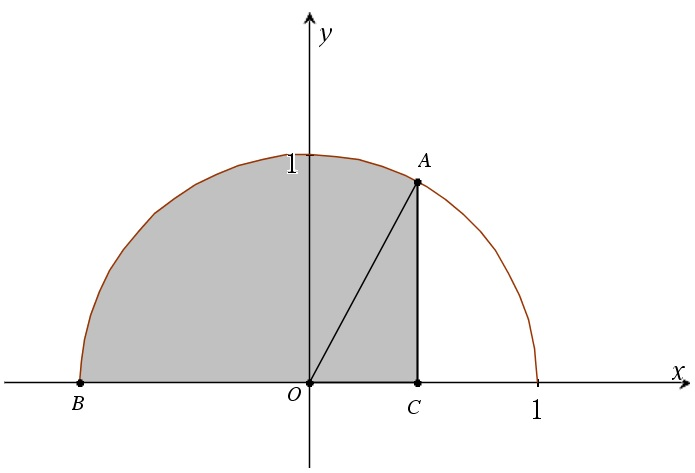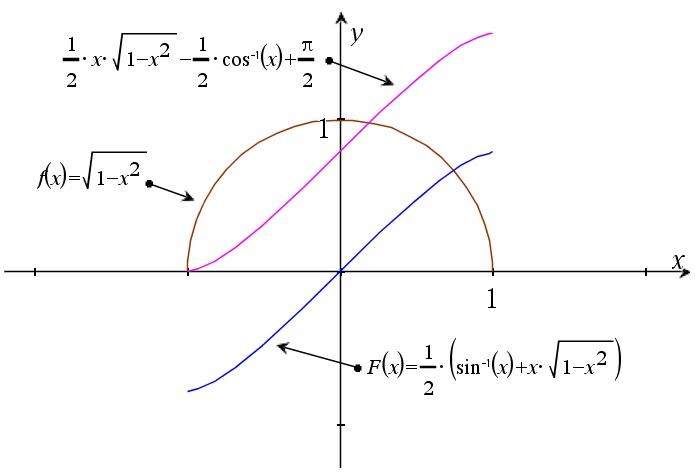# The integral of sqrt(1-x^2) dx

Up a level : Integrals
Previous page : The integral of one over sqrt(x^2+1) dx
Next page : The integral of sqrt(x^2-1) dxNow let us look at$\int {\sqrt {1 - {x^2}} \,dx}$

The integrand is simply describing a half circle with radius one. So we already know$\int\limits_{ - 1}^1 {\sqrt {1 - {x^2}} } dx = \frac{1}{2}\pi \cdot {1^2} = \frac{\pi }{2}$

To find the indefinite integral, let us do the following substitution$\left| \begin{gathered} x = \sin \alpha \hfill \\ dx = \cos \alpha \;d\alpha \hfill \\ \end{gathered} \right.$

We get$\begin{gathered} \int {\sqrt {1 - {x^2}} \,dx} \hfill \\ \quad = \int {\sqrt {1 - {{\sin }^2}\alpha } \cos \alpha \;d\alpha \,} \hfill \\ \quad = \int {{{\cos }^2}\alpha \;dy\,} \hfill \\ \quad = \int {\frac{{1 + \cos 2\alpha }}{2}} \hfill \\ \quad = \frac{1}{2}\int {(1 + \cos 2\alpha )dy} \hfill \\ \quad = \frac{1}{2}\left( {\alpha + \frac{{\sin 2\alpha }}{2}} \right) + C \hfill \\ \quad = \frac{1}{2}\alpha + \frac{1}{4}\sin 2\alpha + C \hfill \\ \end{gathered}$

Here we used a couple of common trig rules. But$\sin 2\alpha = 2\sin \alpha \cos \alpha$

and$x = \sin \alpha \Rightarrow \alpha = {\sin ^{ - 1}}x$

so$\begin{gathered} \sin 2\alpha = 2\sin \alpha \cos \alpha \hfill \\ \quad = 2x\cos {\sin ^{ - 1}}x \hfill \\ \quad = 2x\sqrt {1 - {x^2}} \hfill \\ \end{gathered}$

This gives us$\begin{gathered} \int {\sqrt {1 - {x^2}} \,dx} \hfill \\ \quad = \frac{1}{2}\alpha + \frac{1}{4}\sin 2\alpha + C \hfill \\ \quad = \frac{1}{2}{\sin ^{ - 1}}x + \frac{1}{2}x\sqrt {1 - {x^2}} + C \hfill \\ \quad = \frac{1}{2}({\sin ^{ - 1}}x + x\sqrt {1 - {x^2}} ) \hfill \\ \end{gathered}$

The graph of the integrand vs. the integral is shown below.

We could also find this integral by looking at the half circular disk.If the length OC=x and AC=y we have that the triangle OAC has the area$\frac{1}{2}xy = \frac{1}{2}x\sqrt {1 - {x^2}}$

The area of the sector OAB, if the angle AOB is α, is${\text{Sector OAB = }}\frac{\alpha }{{2\pi }} \cdot \pi = \frac{\alpha }{2}$

But the angle COA=θ is$\theta = {\cos ^{ - 1}}x$

So$\alpha = \pi - \theta$

This means that$\begin{gathered} {\text{Sector OAB = }}\frac{\alpha }{2} = \frac{\pi }{2} - \frac{1}{2}\theta \hfill \\ \quad = \frac{\pi }{2} - \frac{1}{2}{\cos ^{ - 1}}x \hfill \\ \end{gathered}$

So the gray area ABC is$\int\limits_{ - 1}^x {\sqrt {1 - {x^2}} dx = } \frac{1}{2}x\sqrt {1 - {x^2}} + \frac{\pi }{2} - \frac{1}{2}{\cos ^{ - 1}}x$

This means that our primitive function could be written as$\int {\sqrt {1 - {x^2}} \,dx}= \frac{1}{2}x\sqrt {1 - {x^2}} - \frac{1}{2}{\cos ^{ - 1}}x + C$

But that is not what we previously got? But sine and cos are just phase shifted against each other so the inverse should work (as it does). Below is the integrand and the two solutions, with C=π/2 in the second case.Up a level : Integrals
Previous page : The integral of one over sqrt(x^2+1) dx
Next page : The integral of sqrt(x^2-1) dxLast modified: Mar 31, 2023 @ 06:42